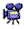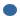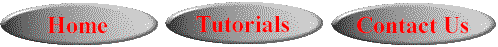# SPSS On-Line Training WorkshopHOME Table of Contents Data Editor Window Syntax  Editor WindowCarl Lee Felix Famoye About Us Chart Editor Window Output Window Overview of Data Analysis Manipulation of Data Analysis of Data Projects & Data Sets Integrate R into SPSS

# Analysis of Data

SPSS consists of many statistical procedures. All of these statistical procedures are under the Analyze menu.  As discussed in the Data Type and Possible Statistical Techniques Section, different data types may require different statistical techniques. In this section, movie clips will be  used to demonstrate some commonly used statistical techniques available in SPSS.

For a general overview of Statistical Procedures in SPSS, watch the movie clip:MOVIE:  Statistical ProceduresClicking on each topic will take you to the page discussing the techniques and the movie clips.Descriptive Statistics includes frequencies, descriptives, explore, crosstabs, ratio, P-P plots and Q-Q plots procedures.Compare Means includes mean procedure, t-test procedure and one-way ANOVA.General Linear Model includes univariate ANOVA procedures, multivariate (MANOVA) procedures, repeated measures ANOVA, and variance components analysis.Generalized Linear Models includes generalized linear models and generalized estimating equations.Mixed Models procedure is an expansion of the general linear model, which allows data to have correlated and non-constant variability (it includes linear mixed models).Correlate includes bivariate and partial correlation, and distance measures.Regression includes linear regression, curve estimation, partial least squares, several types of logistic regression, nonlinear models, two-stage least squares and optimal scalingLoglinear Models includes general loglinear model, logit and model selection.Classification includes two-step clustering, k-mean clustering, hierarchical clustering and discriminant analysis.Data Reduction includes factor analysis, correspondence analysis and optimal scaling.Scale gives reliability analysis and multidimensional scaling.Nonparametric tests include runs, 1-sample K-S, K independent samples, K related samples and others.Time Series includes Time series model creation and application, seasonal decomposition, spectral analysis, autocorrelation and cross-correlation.Survival Models includes life table, Kaplan-Meier model, and Cox regression models.Quality Control includes control charts, Pareto charts and capability analysis.ROC curve for model comparison for categorical response.

In this on-line workshop, you will find many movie clips. Each movie clip will demonstrate some specific usage of SPSS.This online SPSS Training Workshop is developed by Dr Carl Lee, Dr Felix Famoye , student assistants Barbara Shelden and Albert Brown , Department of Mathematics, Central Michigan  University. All rights reserved.